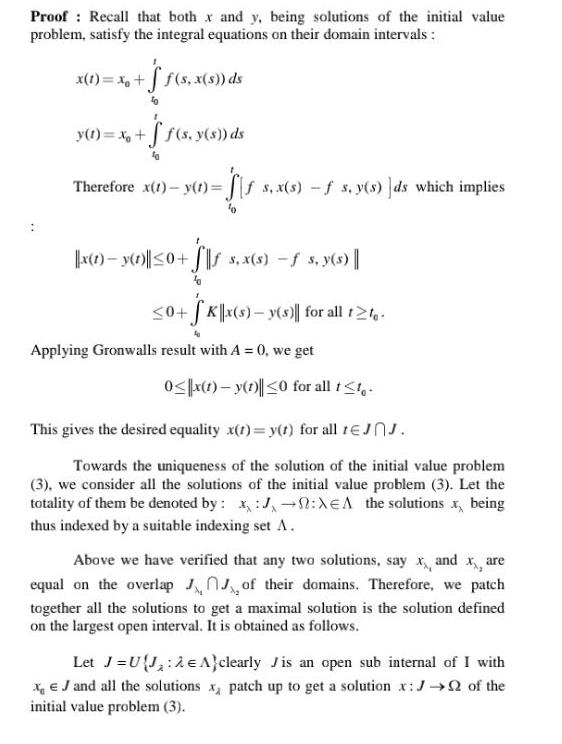Question:

# Proof Recall that both x and y being solutions of the

Last updated: 9/18/2023Proof Recall that both x and y being solutions of the initial value problem satisfy the integral equations on their domain intervals x 1 xo f s x s ds y t x ff s y s ds Therefore x t y t Sis s x s f s y s ds which implies x 1 y 1 0 s x s s y s 0 Kx s y s for all t te Applying Gronwalls result with A 0 we get 0 x 1 y 1 0 for all t t This gives the desired equality x t y t for all tJJ Towards the uniqueness of the solution of the initial value problem 3 we consider all the solutions of the initial value problem 3 Let the totality of them be denoted by x J XEA the solutions x being thus indexed by a suitable indexing set A Above we have verified that any two solutions say x and x are equal on the overlap of their domains Therefore we patch together all the solutions to get a maximal solution is the solution defined on the largest open interval It is obtained as follows Let J U J 2 A clearly Jis an open sub internal of I with XJ and all the solutions x patch up to get a solution x J of the initial value problem 3